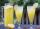# A baker

A baker has 5 1/4 pies in her shop. She cut the pies in pieces that are each 1/8 of a whole pie. How many pieces of pie does she have?

Result

n =  42

#### Solution:

$a = 5 + 1/4 = \dfrac{ 21 }{ 4 } = 5.25 \ \\ b = 1/8 = \dfrac{ 1 }{ 8 } = 0.125 \ \\ \ \\ n = a/b = 5.25/0.125 = 42$

Leave us a comment of this math problem and its solution (i.e. if it is still somewhat unclear...):Be the first to comment!#### Following knowledge from mathematics are needed to solve this word math problem:

Need help calculate sum, simplify or multiply fractions? Try our fraction calculator.

## Next similar math problems:

1. CakesOn the bowl were a few cakes. Jane ate one-third of them, Dana ate a quarter of those cakes that remained. a) What part (of the original number of cakes) Dana ate? b) At least how many cakes could be (initially) on thebowl?
2. ChocolateChildren break chocolate first to third and then every part of another half. What kind got each child? Draw a picture. What part would have received if each piece have halved?
3. Cake 71/3 of a cake shared with 4 people. What share of the whole cake has each people?
4. Pizza 5You have 2/4 of a pizza and you want to share it equally between 2 people how much pizza does each person get?
5. Lengths of the poolMiguel swam 6 lengths of the pool. Mat swam 3 times as far as Miguel. Lionel swam 1/3 as far as Miguel. How many lengths did mat swim?
6. Fractions 4How many 2/3s are in 6?
7. AlmondsRudi has 4 cups of almonds. His trail mix recipe calls for 2/3 cup of almonds. How many batches of trail mix can he make?How many 1/4 cup servings are in 2 and 1/3 cups of lemonade?
9. In fractionsAn ant climbs 2/5 of the pole on the first hour and climbs 1/4 of the pole on the next hour. What part of the pole does the ant climb in two hours?
10. ZdeněkZdeněk picked up 15 l of water from a 100-liter full-water barrel. Write a fraction of what part of Zdeněk's water he picked.
11. Fraction to decimalWrite the fraction 3/22 as a decimal.
12. Product of two fractionsProduct of two fractions is 9 3/5 . If one of the fraction is 9 3/7. Find the other fraction.
13. Fraction and a decimalWrite as a fraction and a decimal. One and two plus three and five hundredths
14. Mixed2improperWrite the mixed number as an improper fraction. 166 2/3
15. Unit rateFind unit rate: 6,840 customers in 45 days
16. Fractions 3Calculate 1/9 of 27:
17. Equation 20In given equation: 8/9-4/5=2/9+x, find x